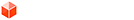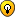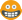## [Code] Print the transformation matrix

### [Code] Print the transformation matrix

Hi, I'm trying to print a transformation matrix, I tried the following:

pointdd = Geom::Point3d.new 11,22,33
tdd = Geom::Transformation.new pointdd
a = tdd.to_a
text="-------------------------------------------------------\n"
4.times do |b|
4.times do |e|
if ((e+1)%4==0)
text << a[e*4+b].to_s+"\n"
else
text << a[e*4+b].to_s+" "+" "+" "+" "+" "+" "+" "+" "+" "+" "
end
end
end
text<<"------------------------------------------------------\n"
UI.messagebox( text, MB_MULTILINE)

I wanted to hear suggestions of more elegant ways to do this. (I wrote several times +" " for failure to escrbir several spaces followed here)

0
Last edited by thomthom on Mon Jun 25, 2012 8:50 am, edited 1 time in total.
Reason: Tagged the thread with [Code]

dacastror

### Re: print the transformation matrix

Code: Select all
`def inspect_transformation(t)    ta = t.to_a    return (0...4).collect() {|i|        ta[i*4, 4].join(', ')    }.join("\n")end`

Code: Select all
`>>> point = Geom::Point3d.new 11,22,33>>> tdd = Geom::Transformation.new pointdd>>> puts inspect_transformation(tdd)1.0, 0.0, 0.0, 0.00.0, 1.0, 0.0, 0.00.0, 0.0, 1.0, 0.011.0, 22.0, 33.0, 1.0`

Edit: And if you like things to line up:
Code: Select all
`def inspect_transformation_adv(t, args={})    format = "%.#{args[:places] || 3}f"    ta = t.to_a.map() {|i|        format%[i]    }    maxlength = ta.max() {|i, j| i.length <=> j.length }.length    ta = ta.map() {|i|        i.ljust(maxlength)    }    return (0...4).collect() {|i|        ta[i*4, 4].join(args[:separator] || ', ')    }.join("\n")end`

Code: Select all
`>>> point = Geom::Point3d.new 11,22,33>>> tdd = Geom::Transformation.new pointdd>>> puts inspect_transformation_adv(tdd)1.000 , 0.000 , 0.000 , 0.000 0.000 , 1.000 , 0.000 , 0.000 0.000 , 0.000 , 1.000 , 0.000 11.000, 22.000, 33.000, 1.000 `

Edit: although that's not the orientation you use
0
Last edited by danielbowring on Mon Jun 25, 2012 7:19 am, edited 1 time in total.danielbowring

### Re: print the transformation matrix

Here's one in your format, although you should probably use one of the ones above (More info here)
Code: Select all
`def inspect_transformation_adv(t, args={})    format = "%.#{args[:places] || 3}f"    ta = t.to_a.map() {|i|        format%[i]    }    maxlength = ta.max() {|i, j| i.length <=> j.length }.length    ta = ta.map() {|i|        i.ljust(maxlength)    }    return (0...4).map() {|i|        (0...4).map() {|j|            ta[j*4+i]        }.join(args[:separator] || ', ')    }.join("\n")end`

Code: Select all
`>>> point = Geom::Point3d.new 11,22,33>>> tdd = Geom::Transformation.new pointdd>>> puts inspect_transformation_adv(tdd)1.000 , 0.000 , 0.000 , 11.0000.000 , 1.000 , 0.000 , 22.0000.000 , 0.000 , 1.000 , 33.0000.000 , 0.000 , 0.000 , 1.000 >>> puts inspect_transformation_adv(t, {:separator => ' '})1.000  0.000  0.000  11.0000.000  1.000  0.000  22.0000.000  0.000  1.000  33.0000.000  0.000  0.000  1.000 `
0danielbowring

### Re: print the transformation matrix

Is it to visually inspect the matrix of entities, if so you can use this tool: viewtopic.php?t=44859
0
Thomas Thomassen — SketchUp Monkey & Coding addictthomthom
PluginStore Author### Re: print the transformation matrix

Here's my simple brute force method:

Code: Select all
`  def trans_matrix_text( gt )    return "Not a trnasformation object!" unless gt.is_a?(Geom::Transformation)        a = gt.to_a        w = 3  # width    a.each{|n| s = n.to_s.size; w = s if s>w }    l = (w*4)+3        # create a line output format string:    fmt = "  | %#{w}s %#{w}s %#{w}s %#{w}s |\n"        text = "\n"        text << (32.chr*2)<<151.chr<<151.chr<<(32.chr*l)<<151.chr<<151.chr<<"\n"    text << fmt % [  a.to_s,  a.to_s,   a.to_s,  a.to_s ]    text << fmt % [  a.to_s,  a.to_s,   a.to_s,  a.to_s ]    text << fmt % [  a.to_s,  a.to_s,  a.to_s, a.to_s ]    text << fmt % [ a.to_s, a.to_s,  a.to_s, a.to_s ]    text << (32.chr*2)<<151.chr<<151.chr<<(32.chr*l)<<151.chr<<151.chr<<"\n"        return text  end # def  pt = Geom::Point3d.new( 11,22,33 )  text = trans_matrix_text( Geom::Transformation.new(pt) )    UI.messagebox( text, MB_MULTILINE, "Tranformation Matrix" )`

I have tweaked my system by copying Consolas font to the font used by dialogs, so I have monospaced text output. (It was easier than tweaking the resources in the executable after each update.)

trans_matrix_MB.png

trans_matrix_IO.png0
I'm not here much anymore. But a PM will fire email notifications.Dan Rathbun
PluginStore Author### Re: [Code] Print the transformation matrix

Thanks for responding, I have helped a lot.me a question arises about the transformations. if I need to apply a transformation to an object but not in the principal coordinate axes, but the coordinate axes of a group (rotated) what should I use?

0

dacastror

### Re: [Code] Print the transformation matrix

You mean, transform an object around its own axis and not world axis?
0
Thomas Thomassen — SketchUp Monkey & Coding addictthomthom
PluginStore Author### Re: [Code] Print the transformation matrix

thomthom wrote:You mean, transform an object around its own axis and not world axis?

yes, exactly
0

dacastror

### Re: [Code] Print the transformation matrix

Create a local transformation and apply with with .transform!( tramsformation ) instead of setting the .transformation matrix manually. Or am I missing something?
0
Thomas Thomassen — SketchUp Monkey & Coding addictthomthom
PluginStore Author### Re: [Code] Print the transformation matrix

thomthom wrote:Create a local transformation and apply with with .transform!( tramsformation ) instead of setting the .transformation matrix manually. Or am I missing something?

How to create a local transformation? I would like to see some sample piece of code to guide me
0

dacastror

### Re: [Code] Print the transformation matrix

I wonder if there is any way to know the orientation of a group with respect to the axis of the world
0

dacastror

### Re: [Code] Print the transformation matrix

You have the model's axes from X_AXIS etc and ORIGIN.
Then you have group.transformation.axes or group.transformation.xaxis etc... and group.transformation.origin too...
So you can find the relative locations [which are points3ds] and angles between axes [which are vector3ds] - see the methods for those types...
0
TIGTIG
Global Moderator

### Re: [Code] Print the transformation matrix

dacastror wrote:
thomthom wrote:Create a local transformation and apply with with .transform!( tramsformation ) instead of setting the .transformation matrix manually. Or am I missing something?

How to create a local transformation? I would like to see some sample piece of code to guide me

Code: Select all
`point = instance.transformation.originvector = instance.transformation.yaxisrotation = Geom::Transformation.rotation( point, vector, 30.degrees )instance.transform!( rotation ) `
0
Thomas Thomassen — SketchUp Monkey & Coding addictthomthom
PluginStore Author### Re: [Code] Print the transformation matrix

thank you very much Thomas and Tig that prompt reply!I will try to implement your suggestions right now
0

dacastror